# Difference between revisions of "SMHS EDA"

## Scientific Methods for Health Sciences - Exploratory Data Analysis (EDA), Charts and Plots

### Overview

• What is data? Data is a collection of facts, observations or information, such as values or measurements. Data can be numbers, measurements, or even just description of things (meta-data). Data types can be divided into two big categories of quantitative (numerical information) and qualitative data (descriptive information).
• Quantitative data is anything that can be quantified or expressed as a number. For example, the scores on a math test and the weight of girls in a fourth grade class are both quantitative data. This form of data is often referred to as the measurable data, and it allows scientists to perform various arithmetic operations, such as addition, multiplication, or functional-evaluation. It also allows scientists to find the parameters of a population. There are two major types of quantitative data: discrete and continuous.
• Discrete quantitative data results from a finite (or infinite but countable) number of possible values that are present in a given data set, and the values of this data type can constitute a sequence of isolated or separated points on the real number line.
• Continuous quantitative data results from infinite and dense possible values that the observations can take.
• Qualitative data cannot be expressed as numbers. Examples of qualitative data elements include gender, ethnicity or religious preference. Categorical data (qualitative or nominal) results from placing individuals into groups or categories. Ordinal and qualitative categorical data types both fall into this category.

In statistics, exploratory data analysis (EDA) is an approach to analyze data sets to summarize their main characteristics. Modern statistics regards the graphical visualization and interrogation of data as a critical component of any reliable method for statistical modeling, analysis and interpretation of data. Formally, there are two types of data analysis that should be employed in concert on the same set of data to make a valid and robust inference: graphical techniques and quantitative techniques. We will discuss many of these later, but below is a snapshot of EDA approaches:

• Box plot, Histogram; Multi-vari chart; Run chart; Pareto chart; Scatter plot; Stem-and-leaf plot
• Parallel coordinates; Odds ratio; Multidimensional scaling; Targeted projection pursuit; Principal component analysis; Multi-linear PCA; Projection methods such as grand tour, guided tour and manual tour
• Median polish, Trimean

### Motivation

The feel of data comes clearly from the application of various graphical techniques, which serves as a perfect window to human perspective and sense. The primary goal of EDA is to maximize the analyst’s insight into a data set and into the underlying structure thereof. To get a feel for the data, it is not enough for the analyst to know what is in it; he or she must also know what is not in the data, and the only way to do this is to draw on our own pattern recognition and comparative abilities in the context of a series of judicious graphical techniques applied to the data. The main objectives of EDA are to:

• Suggest hypotheses about the causes of observed phenomena
• Assess (parametric) assumptions on which statistical inference will be based;
• Support the selection of appropriate statistical tools and techniques
• Provide a basis for further data collection through surveys and experiments

### Theory

Many EDA techniques have been proposed, validated and adopted for various statistical methodologies. Here is an introduction to some of the most frequently used EDA charts and quantitative techniques.

#### Box-and-Whisker plot

The Box-and-Whisker plot is an efficient way for presenting data, especially when it comes to comparing multiple groups thereof. In the box plot, we can mark-off the five-number summary of a data set (minimum, 25th percentile, median, 75th percentile, maximum). The box contains 50% of the data, and the upper edge of the box represents the 75th percentile, while the lower edge is the 25th percentile. The median is represented by a line drawn in the middle of the box. If the median is not in the middle, then the data are skewed. The ends of the lines (or "whiskers") represent the minimum and maximum values of the data set, unless there are outliers. Outliers are observations below $$Q_1-1.5(IQR)$$ or above $$Q_3+1.5(IQR)$$, where $$Q_1$$ is the 25th percentile, $$Q_3$$ is the 75th percentile, and $$IQR=Q_3-Q_1$$ (the interquartile range). The advantage of a box plot is that it provides graphically the location and the spread of the data set, as well as an idea about the skewness of the data and a comparison between variables by constructing side-by-side box plots.#### Histogram

Histograms represent a graphical visualization of tabulated frequencies or counts of data within equally-spaced partitions of the data range. It shows the proportion of measurements that fall into each of the categories defined by the partition of the data range space.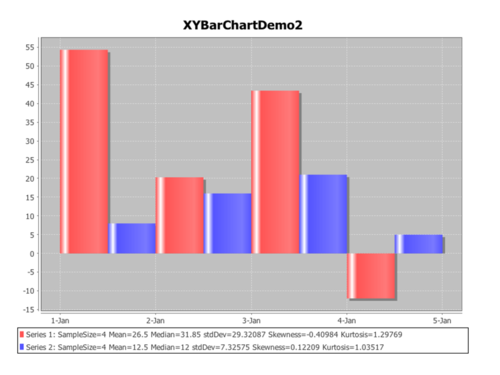• Comment: When comparing the two series from the histogram above, we can easily tell that the pattern of Series 2 is more obvious compared to that of Series 1. Our intuition may come from the fact that Series 1 has more extreme values across five days, with the values for Jan 1st and Jan 3rd being extremely high (almost 55 for Jan 1st), while that for Jan 4th is almost -12. However, the values for Series 2 are all above 0 and fluctuate between 5 and 20.

#### Dot Chart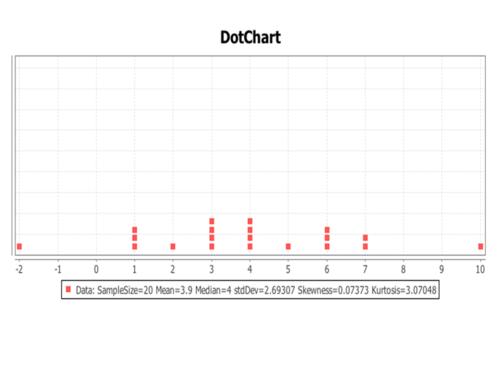• Comment: The dot chart above gives a clear picture of the values of all the data points and makes the fundamental measurements easily readable. We can tell that most of the values of the data fluctuate between 1 and 7 with a mean of 3.9 and a median of 4. There are two obvious outliers valued -2 and 10.

#### Scatter Plot

Scatter plots use Cartesian coordinates to display values for two variables from a set of data, which is displayed as a collection of points. The values of these variables are determined by the position on the horizontal and vertical axes.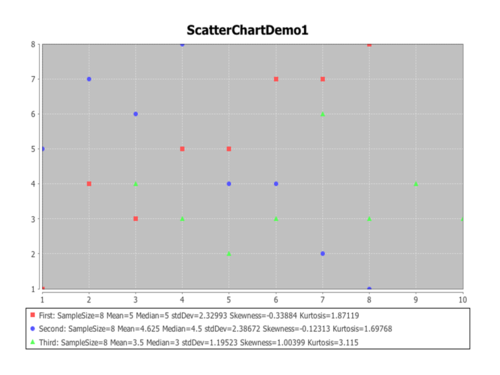• Comment: The X- and Y-axes display values for two variables, and all the data points drawn in the chart are coordinates indicating a pair of values for both variables.
• For the first series, all the data points lie on and above the diagonal line; so each Y variable increases faster or equal to the X variable with which it is paired. We can therefore infer a positive linear association between X and Y.
• For the second series, most data are located along the line except for two outliers (4,8) and (1,5). So for most of the data points, each Y variable decreases slower or equal to the X variable with which it is paired. We may therefore infer a negative linear association between X and Y.
• For the third series, we can’t draw a line association between X and Y; instead, a quadratic pattern would work better here.

#### QQ Plot

In Quantile-Quantile plots, the observed values are plotted against theoretical quantiles in QQ charts. A line of good fit is drawn to show the behavior of the data values against the theoretical distribution. If F() is a cumulative distribution function, then a quantile (q) - which is also known as a percentile - is defined as a solution to the equation $$F(q)=p$$,that is $$q=F^{-1}(p)$$.

• Comment: From the chart above, we can see that the data follows a normal distribution in general given all the data points (noted in red) located alongside the line. However, the data doesn’t follow a normal distribution tightly because there are data points located pretty far from the line. We can also infer that the sampled data may not be representative enough of the population because of the limited size of the sample.

#### Median polish

Median polish is an EDA procedure proposed by John Tukey. It finds an additively fit model for data in a two-way layout table of the form row effect + column effect + overall mean. It is an iterative algorithm for removing any trends by computing medians for various coordinates on the spatial domain D.

#### Trimean

Trimean is a measure of a probability distribution’s location, which is defined as a weighted average of the distribution’s median and its two quartiles. It combines the median’s emphasis on center values with the midhinge’s attention to the extremes. It is a remarkably efficient estimator of population mean, especially for large data sets (consisting of more than 100 points, for example) from a symmetric population.

$$\frac{Q_1+2Q_2+Q_3}{4}$$

### Applications

• This article provides a thorough introduction to EDA, for it discusses the basic concepts, objectives, and techniques with which EDA is associated. It also includes case studies in which EDA is applied. The case studies include eight different types of charts for univariate analyses, and they also introduce the concepts of reliability and multi-factor studies. The article gives specific examples with background, thereby demonstrating how output and interpretations of results are useful resources for learning EDA.
• This article begins with a general introduction to data analysis and explains EDA via examples that employ various graphical analyses. It also serves as a basic and general introduction to the concepts associated with EDA and is a good starting place for studying these concepts.
• The SOCR Motion Charts Project enables complex data visualization, see the SOCR MotionChart webapp. The SOCR Motion Charts provide an interactive infrastructure for discovery-based exploratory analysis of multivariate data.
• Suppose we want to explore the relationship between two variables in the dataset UR (Unemployment Rate) and HPI (Housing Price Index) in the state of Alabama over 2000 to 2006. First, how does the UR in Alabama change from 2000 to 2006?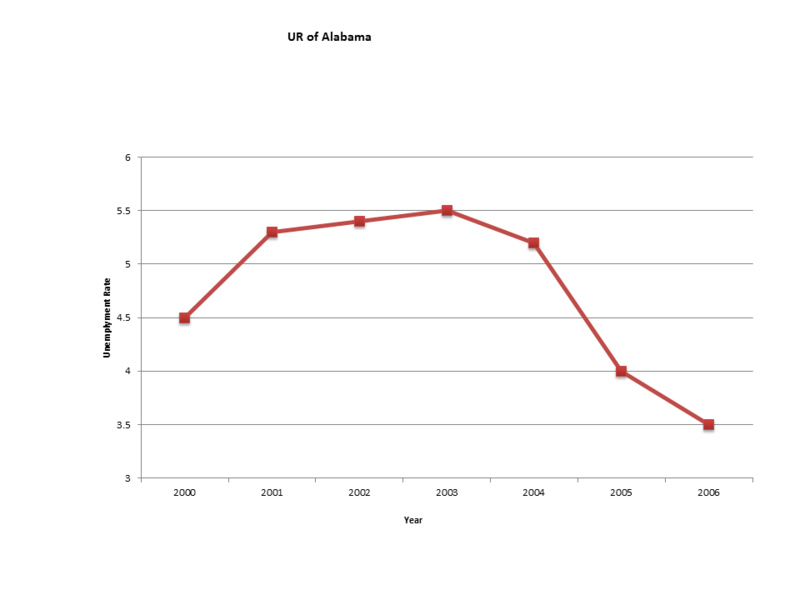From this chart, we can see that the UR in Alabama increases from 2000 to 2003, then decreases sharply from 2004 to 2006. What is the UR for other states over the same period?All the states appear to follow similar patterns. Now, let’s study relationships between UR and HPI in a single state - say, Alabama - across this time span.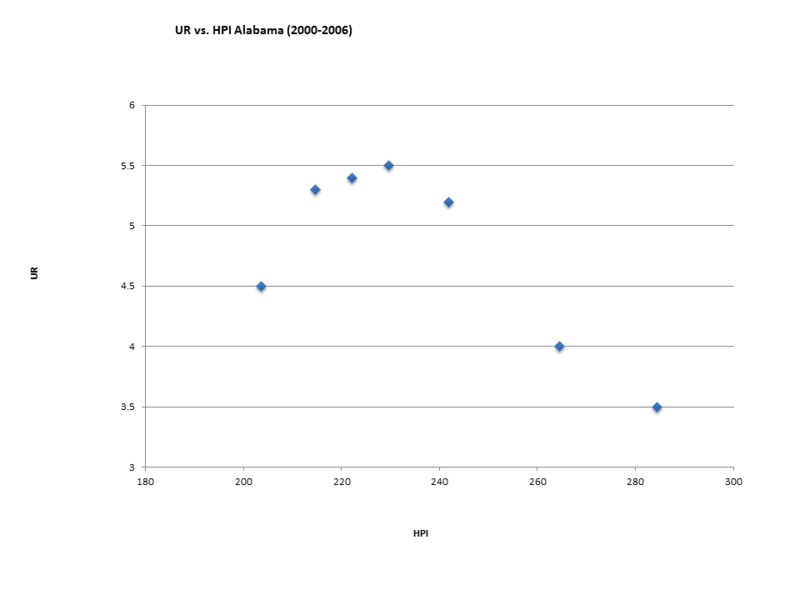The chart above suggests that HPI increases through time in Alabama, while UR increases at first and then exhibits a sharp drop between 2004 and 2006. If there is any association between UR and HPI, it appears to be quadratic rather than linear. Similarly, if we extend the graph to the three states from different regions, we generate the following chart: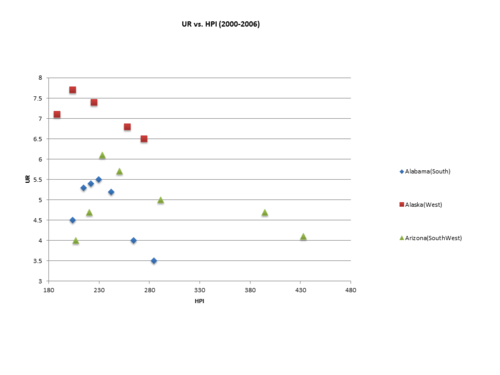We can now address the question: is there any association between UR and HPI among all the states based on the chart? The motion chart, however, makes the study much more interesting by exhibiting a moving chart with UR vs. HPI of all 50 states during the period from 2000 to 2006. This allows us to get an idea of the changing values over the years among all states. You’re welcome to play with the data to see how the chart changes using the link listed above.

#### Mixed quantitative and qualitative methods

Suppose we want to design and complete a comparative longitudinal study using mixed methods to examine an intervention for recurrent depression. We want to use 2 patient groups for the real intervention and 2 groups with a sham intervention, each meeting once a week for three weeks. To determine if the intervention is effective, we plan to collect data pre- and post-intervention on 10 variables such as self-efficacy, social support, quality of life, depression rating, etc. at 4 time points (baseline, prior to commencing the study, at the conclusion of the 3 weeks of intervention, 3 months out and chronically, 6 months after the completion of the study).

Mixed methods for combining quantitative with qualitative data analyses may be used in such cases. These techniques contextualize quantitative statistical inference with methods from the qualitative interviewing process. Our primary analytical focus is to obtain inferences regarding factors (e.g., subject phenotypes) that may be associated with observed outcomes (e.g., depression) and treatments (e.g., intervention/sham). The statistical inference in this context involves conclusions about the entire study population and stratified cohorts based on various trait characteristics. Exploratory and graphical summaries of relevant numerical data may support such quantitative statistical inference. Both statistical significances (e.g., effect-sizes) and clinical differences (e.g., depression scale rating) in parent-related outcomes may be studied continuously starting at baseline (before intervention) to the chronic state (6 months after intervention). For instance, when examining continuous variables, an effect size may be calculated and assessed using Cohen's d. Effect sizes of 0.1–0.3 are generally considered small, whereas effect sizes of 0.3–0.5 and ≥ 0.5 are considered to be medium and large, respectively. For secondary outcomes, such as dichotomized adherence, the odds ratios (ORs) may be calculated to report the effect size. The OR is the ratio of the odds of an outcome (e.g., being adherent) of one group (e.g., baseline) to the odds of the outcome of another group (chronic, post 6th month follow-up). OR-derived effect-sizes may be classified as small ($1.5<OR<2.5$), medium ($2.5<OR<4.3$), or high ($OR>4.3$). Frequency and percentage representation of nominal categorical data will be used and medians and quartiles (Q1–Q3) may be calculated for continuous, non-normally distributed variables.

### Problems

• Two random samples were taken to determine backpack load difference between seniors and freshmen, in pounds. The following are the summaries:
 Year Mean SD Median Min Max Range Count Freshmen 20.43 4.21 17.2 5.78 31.68 25.9 115 Senior 18.67 4.21 18.67 5.31 27.66 22.35 157
• Which of the following plots would be the most useful in comparing the two sets of backpack weights? Choose One Answer:
(a) Histograms
(b) Dot Plots
(c) Scatter Plots
(d) Box Plots
• School administrators are interested in examining the relationship between height and GPA. What type of plot should they use to display this relationship? Choose one answer.
(a) box plot
(b) scatter plot
(c) line plot
(d) dot plot
• What would be the most appropriate plot for comparing the heights of the 8th graders from different ethnic backgrounds? Choose one answer.
(a) bar charts
(b) side by side boxplot
(c) histograms
(d) pie charts
• There is a company in which a very small minority of males (3%) receives three times the median salary of all males, and a very small minority of females (3%) receives one-third of the median salary of all females. What do you expect the side-by-side boxplot of male and female salaries to look like? Choose one answer.
(a) Both boxplots will be skewed and the median line will not be in the middle of any of the boxes.
(b) Both boxplots will be skewed, in the case of the females the median line will be close to the top of the box and in the case of the males the median line will be closer to the bottom of the box.
(c) Need to have the actual data to compare the shape of the boxplots.
(d) Both boxplots will be skewed, in the case of males the median line will be close to the top of the box and for the females the median line will be closer to the bottom of the box.
• A researcher has collected the following information on a random sample of 200 adults in the 40-50 age range: Weight in pounds Heart beats per minute Smoker or non-smoker Single or married

He wants to examine the relationship between: 1) heart beat per minute and weight, and 2) smoking and marital status. Choose one answer.

(a) He should draw a scatter plot of heart beat and weight, and a segmented bar chart of smoking and marital status.
(b) He should draw a side by side boxplot of heart beat and weight and a scatterplot of smoking and marital status.
(c) He should draw a side by side boxplot of smoking and marital status and a segmented bar chart of hear beat and weight.
(d) He should draw a back to back stem and leaf plot of weight and heart beat and examine the cell frequencies in the contingency table for smoking by marital status.
• As part of an experiment in perception, 160 University of Michigan psych students completed a task on identifying similar objects. On average, the students spent 8.25 minutes with sa tandard deviation of 2.4 minutes. However, the minimum time was 2.3 minutes and one students worked for almost 60 minutes. What is the best description of the histogram of times that students spent on this task? Choose one answer.
(a) The histogram of times could be symmetrical and not normal with major outliers.
(b) The histogram of times could be left skewed, and in case there are any outliers, it is likely that they will be smaller than the mean.
(c) The histogram of times could be right skewed, and in the case of any outliers, it is likely that they will be larger than the mean.
(d) The histogram of times could be normal with no major outliers.